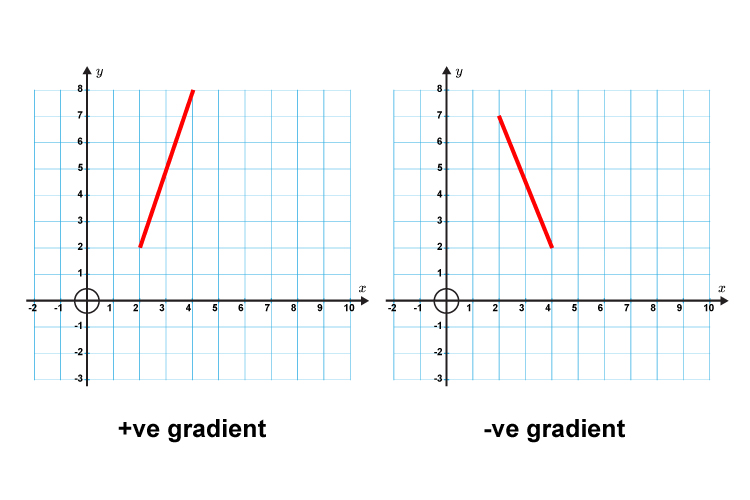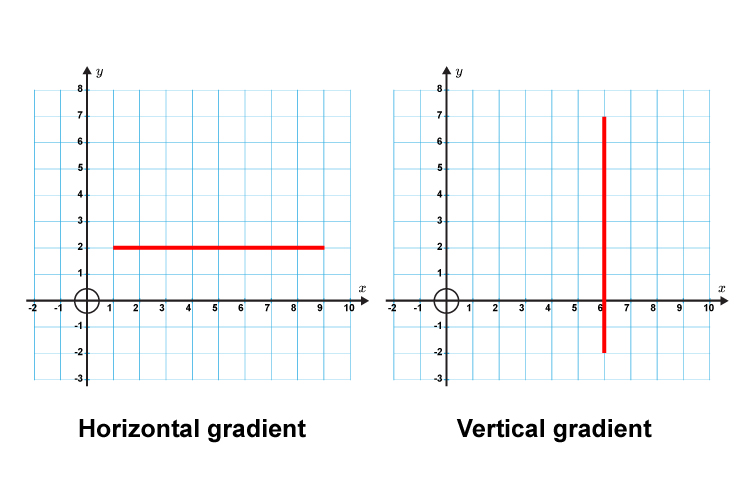We know that:But what are the gradients of the following graphs?We can work it out as follows:

## Horizontal line

Gradient(m)=(y(chang\e\ i\n))/(x(chang\e\ i\n)

If the line is horizontal then:

(m)=(zero)/(It\ cou\ld\ be\ 5,\or\6\or7\ or\ any\ n\umber

Zero divided by any number is zero.

(Try zero divided by any number on your calculator).

So the slope of a horizontal line is zero.

## Vertical line

G(m)=(y(chang\e\ i\n))/(x(chang\e\ i\n)

If the line is vertical then:

G(m)=(It\ cou\ld\ be\ 5,\or\6\or7\ or\ any\ n\umber)/(zero)

Any number divided by zero is either undefined (i.e. can’t do it), has no slope, or is an infinite number.

(Try a number divided by zero on your calculator).

So the slope of a vertical line is undefined, has no slope, or is infinite.

A vertical line has no gradient.

If you are unsure, your calculator will always tell you.Your calculator will tell you that a number divided by zero is ERROR.